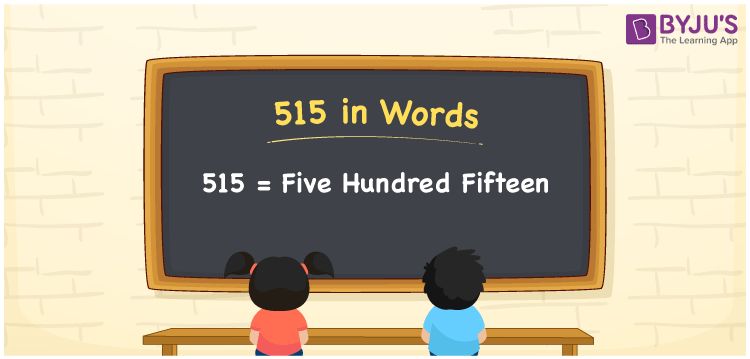# 515 in Words

515 in words is written as Five Hundred Fifteen. The name of number 515 in English is “Five Hundred Fifteen”. The word Five Hundred Fifteen is used in many real-life situations. For example, there are Five Hundred Fifteen students in a classroom. Thus, it is a counting number. Also, to represent the currency equal to 515, we can write it in words as Rupees Five Hundred Fifteen or Five Hundred Fifteen rupees. Therefore, it is necessary to learn the numbers in words, for the ease of understanding and expressing them.

 515 in words Five Hundred Fifteen Five Hundred Fifteen in Numbers 515

## 515 in English Words## How to Write 515 in Words?

If we know the place value of digits of 515, then we can easily express it in words. The place value is basically the position of a digit in a number. 515 is a three-digit number, therefore, we can specify the position of each digit of 515 in a place value chart. In the Indian numbering system, the order of place value of digits from right to left is given by:

 Hundreds Tens Ones 5 1 5

We can write it in expanded form as:

5 × Hundred + 1 × Ten + 5 × One

= 5 × 100 + 1 × 10 + 5 × 1

= 500 + 10 + 5

= 515

= Five Hundred Fifteen

Therefore, 515 in words is written as eight hundred thirties.

515 is a natural number that precedes 516 and succeeds 514.

515 in words – Five Hundred Fifteen

Is 515 an odd number? – Yes

Is 515 an even number? – No

Is 515 a perfect square number? – No

Is 515 a perfect cube number? – No

Is 515 a prime number? – No

Is 515 a composite number? – Yes

## Frequently Asked Questions on 515 in Words

Q1

### How to write 515 in words?

515 in English is written as “Five Hundred Fifteen”.
Q2

### Is 515 an odd number or even number?

515 is an odd number because it is not wholly divisible by 2.
Q3

### Write Five Hundred Fifteen in numbers.

Five Hundred Fifteen in numbers is 515.